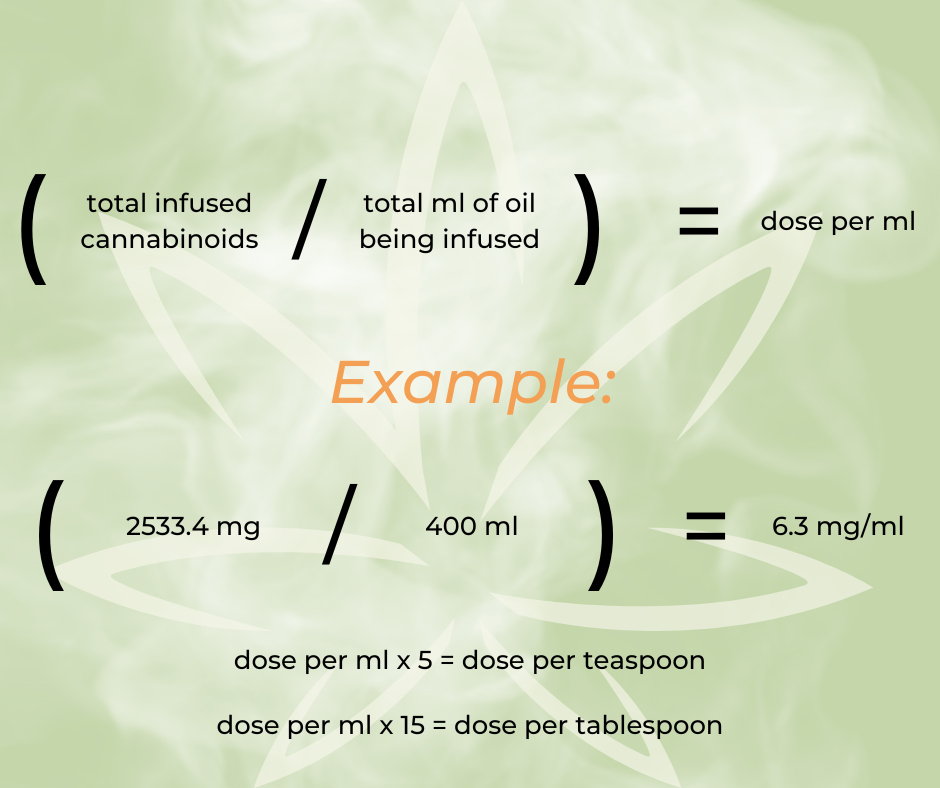top of page
• Lisa Capitani RN

# Math is... fun?This Sunday (May 21, 2023), I'll be speaking a my FIRST big event! NECANN Hartford, CT at the XL Center. I am posting this blog before my talk on DIY Plant Medicine "Best Practices" because doing the math was the hardest (and most boring) part of learning to make my own medicine. I don't want to full of my speaking time with math (eew) so this post will help all my inspired and empowered listeners get their DIY practice off the ground!

Remember to be kind to yourself, ask for help, learn from mistakes, and HAVE FUN!

The basic math to calculate decarboxylation loss is:Or plug your values into this calculator:

decarb loss
.xlsx

The basic math to calculate infusion loss is:Or use the calculator:

Infusion loss
.xlsx

Once you have this quantity (number of usable mg) you can determine very easily what your dose by volume would be by doing the following:To make your life easier- here's the calculator I use when planning my infusions. Plug your known values into the orange boxes and let the calculator do the rest. You need to know:

1. How many mg/ml you want your end product to be

2. What the % acid form of the cannabinoids in your product is (i.e. 25% THCA- this is why you need COAs!)

3. What volume of oil you want to infuse (in ml)

4. The infusion rate for the oil (most are around 80%; do your research or use this rate in a pinch)

The weight needed of each raw form will populate in the green squares when you've entered all the information needed!

Infusion Calculator
.xlsx

To calculate gummy doses using RSO/HPO, I use the following simple formula:or this calculator:

Gummy calculator
.xlsx